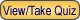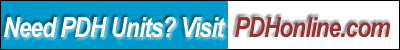Print this page

Course Description

Mathcad is a powerful tool for solving and visualizing mathematical and engineering problems. Its flexible calculation and documentation environment is recognized as the easiest to use GUI-based math software to document and compute an engineering problem with mathematical notation. As the second generation of Mathcad, Mathcad Prime is more intuitive and easier to use than its predecessor. It provides verification, validation, documentation and re-use of engineering calculations. In this lesson, you will learn the new features and functions of Mathcad Prime, including ribbon-style interface, symbolic processors and spec tables. This course includes a multiple choice quiz at the end.

Learning Objective

At the conclusion of this course, the student will:

• Be familiar with the interface, graphics, text and computational features of Mathcad Prime;
• Be able to perform engineering calculations using Mathcad Prime;
• Be able to minimize calculation errors; and
• Be able to produce quality engineering documentations.

Course Introduction

At the time of its first release in 1986, Mathcad by MathSoft was the first computer software to introduce live editing typeset mathematical notation, automatic computation and engineering unit error checking. Since then, Mathcad has gone through many upgrades and improvements, and has become a valuable technical computing tool for engineers and architects.

In 2006, the Parametric Technology Corporation (PTC) acquired MathSoft. The following year PTC released Mathcad 14.0, which offered several significant enhancements. Mathcad 15.0, released in 2010, also provided many improved and updated functions. After Mathcad 15.0, PTC decided to go in an entirely new direction with Mathcad Prime 1.0 (2011) and Mathcad Prime 2.0 (2012). Some of the new features include new Prime User Interface (UI) with snap-to-grid workspace, multiple document interface, new ribbon menus and status bars, new matrix navigation/view, plots and matrices support units, and new label styles.

Worksheets from earlier versions of Mathcad cannot be opened directly into Mathcad Prime 2.0. Instead PTC provides an XMCD, MCD Converter that can run in batch mode to convert many files at once or to convert an individual worksheet. If you want to convert worksheets from previous versions of Mathcad, you need to download PTC Mathcad 15 Free Trial. The converter requires Mathcad 15.0 to be installed so that you can convert any worksheets from past versions of Mathcad.

Course Content

Mathcad provides a rich problem-solving environment that gives you a wide variety of analysis and visualization tools. Unlike other technical software, Mathcad does math the same way you do. That is because it looks and works like a scratchpad and pencil. Mathcad's on-screen interface is a blank worksheet on which you can enter equations, graph data or functions, and annotate with text - anywhere on the worksheet. Some of the important computational features of Mathcad for engineering applications are:

• Built-in units of measurement and dimension checking.
• Built-in solver for simultaneous equations and inequalities. Summations, products and iteration. Trigonometric, hyperbolic, exponential and Bessel functions. Fast Fourier transformation, both one and two dimensional. User-defined functions.
• Vectors and matrices, including operation for matrix multiplication, matrix inverse, tranpose, determinant, dot and cross product.

Mathematical expressions in Mathcad look the way you would see them in a textbook or a reference book. The only difference is that Mathcad's equations and graphs are live, which means Mathcad recalculates the math and redraws the graphs every time you change any data, variable, or equation in a worksheet. Please click on the following weblink to learn more about the features and functions of Mathcad Prime 2.0:

(PDF)

To learn the basic features of Mathcad Prime, you need to have at least a copy of Mathcad Prime Express, which is free and available from the PTC website. Once you install the software, you need to study the Mathcad Prime's built-in tutorial. In particular, you need to learn how to

1. enter text
2. build mathematical expressions
3. define variables
4. define functions
5. define range variables
6. create graphs
7. specify units
You may also access the free Mathcad Prime Tutorial on PTC University Learning Exchange.

In addition, you need to watch the following video lessons to learn navigation, text handling, calculation, how to use units in calculations, how to use variables and functions, how to work with data, and how to create engineering worksheets and lab reports:

1. Mathcad Prime 1.0 Demo (11:26 video)
2. Mathcad Prime 2.0 Webinar (1:00:15 video)
3. Mathcad Basics Webinar: Getting Started (24:04 video)
4. Mathcad Basics Webinar: Worksheet Creation and Calculation Management (21:42 video)
5. Mathcad Basics Webinar: Computer Algebra and Solve Blocks (19:57 video)
6. Mathcad Basics Webinars: Working with Data (Part 1 - Entering Data) (11:10 video)
7. Mathcad Basics Webinars: Working with Data (Part 2 - Entering Data) (16:35 video)
8. Practice in Mathcad Prime 2.0 (1:19:41 video)

Furthermore, you need to download and study the following two Mathcad application files since you will need them to solve several problems when taking the quiz.

1. Section Properties of Uneven I Beam (Uneven-I-Beam.mcdx, Mathcad Prime 2.0, 33 kb)
2. Spring Force Calculator (Spring-Force-Calculator.mcdx, Mathcad Prime 2.0, 70 kb)

Course Summary

This course covers some of the basic features of Mathcad Prime, which include interface features, graphical features, computational features, and text and document features. Through this online course, the student should be able to use Mathcad Prime to solve some of the routine design problems in his/her design practice. The technical documents prepared using Mathcad can be easily understood and verified by others. In addition, using Mathcad in your work can relieve you from tedious and repetitive design calculations, eliminate arithmetic errors, and produce quality design calculations.